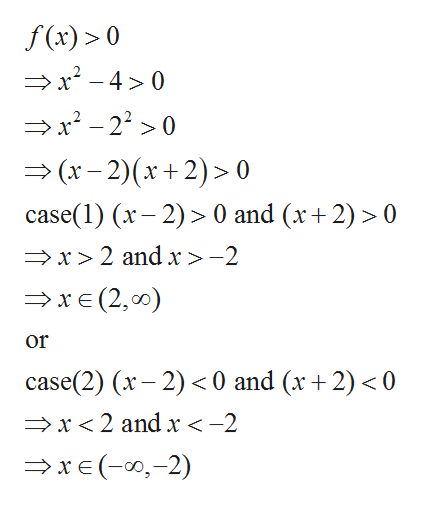# Use the function f(x)=x^2-4 and g(x)=2x+20 answer parts (a)-(g)(a) Solve f(x)>0.What is the correct answer in interval notation?

Question
2 views

Use the function f(x)=x^2-4 and g(x)=2x+20 answer parts (a)-(g)

(a) Solve f(x)>0.

What is the correct answer in interval notation?

check_circle

Step 1

Given function is

Step 2

On solving for f ...help_outlineImage Transcriptionclosef(x)> 0 x2-40 x-220 (x-2)(x +2)> 0 case(1) (x2)0 and (x2)> 0 x2 and x>-2 xE (2, 00) or case(2) (x 2) 0 and (x+2)<0 x2 and x < -2 xe(2) fullscreen

### Want to see the full answer?

See Solution

#### Want to see this answer and more?

Solutions are written by subject experts who are available 24/7. Questions are typically answered within 1 hour.*

See Solution
*Response times may vary by subject and question.
Tagged in

### Other